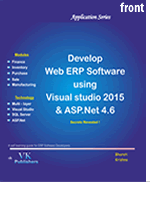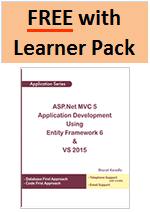# Visual Basic 2005 Type Conversion Functions

Sometimes we need to change the type of a variable as the program proceeds. Many a times the compiler itself effects the change. In some cases, we need to explicitly effect the change. The former type of conversion is referred to as implicit conversion. The later type is referred to as explicit conversion. Implicit conversions happen when the change effected does not change the value of the variable and there is no loss of data. Explicit conversions are a must when the change is from a large type to a smaller type.

### Conversions Between Strings and Other Types

We can convert a numeric, Boolean, or date/time value to a String. We can also convert in the reverse direction - from a string value to numeric, Boolean, or Date - provided the contents of the string can be interpreted as a valid value of the destination data type. If they cannot, a run-time error occurs.

### Conversion of Numbers to Strings

We can use the Format function to convert a number to a formatted string, which can include not only the appropriate digits but also formatting symbols such as a currency sign (such as \$), thousands separators or digit grouping symbols (such as ,), and a decimal separator (such as .). The Format function automatically uses the appropriate symbols according to the Regional Options settings specified in the Windows Control Panel.

Note that the concatenation (&) operator can convert a number to a string implicitly, as the following example shows.

The following statement converts count to a String value.
```Str = "The total iterations are " & count
```

### Conversion of Strings to Numbers

We can use the Val function to explicitly convert the digits in a string to a number. Val reads the string until it encounters a character other than a digit, space, tab, line feed, or period. Val converts all appropriate characters to a numeric value. For example, the following statement returns the value 141.825.

Val(" 14 1.825 metres")

When Visual Basic converts a string to a numeric value, it uses the Regional Options settings specified in the Windows Control Panel to interpret the thousands separator, decimal separator, and currency symbol. Below are the list of fumctions and its conversions.
```FUNCTION 					CONVERTS ITS ARGUMENT To

CBool 						Boolean
CByte						Byte
CChar 						Unicode character
CDate 						Date
CDb1 						Double
CDec 						Decimal
Clnt						integer (4-byte integer, 1nt32)
CLng 						1ong (8-byte integer, 1nt64)
CObj 						Object
CShort 						Short (2-byte integer, Inti6)
CSng 						Single
CStr 						String
```

To convert the variable initialized as Integer to a double, use the function CDb1.

```Dim Amount As Integer
Dim dblAmount As Doube
dblAmount = CDb1(Amount)

Suppose we have declared two integers, as follows.

Dim A As Integer, B As Integer
A =23
B =7
```

The result of the operation A / B will be a double value, the following statement

Console.Write(A / B)

displays the value 3.28571428571429. The result is a double, which provides the greatest possible accuracy. If we attempt to assign the result to a variable that hasn't been declared as Double, and the Strict option is On, then VB.NET will generate an error message. No other data type can accept this value without loss of accuracy.

As a reminder, the Short data type is equivalent to the old Integer type, and the CShort( ) function converts its argument to an Intl6 value. The Integer data type is represented by 4 bytes (32 bits), and to convert a value to 1nt32 type, use the CLnt() function. Finally, the CLng() function converts its argument to an 1nt64 value.

We can also use the CType() function to convert a variable or expression from one type to another. Let's say the variable A has been declared as String and holds the value "34.56" The following statement converts the value of the A variable to a Decimal value and uses it in a calculation:

Dim A As String "34.56"
Dim B As Double
B = CType(A, Double) / 1.14

The conversion is necessary only if the Strict option is On, but it's a good practice to perform your conversions explicitly.

Let's say we have declared and initialized two variables, an integer and double, with the following statements.

Dim totoal As Integer=99
Dim pi As Double=3.1415926535897931

If the Strict option is On and we attempt to assign the value of the pi variable to the count variable, the compiler will generate an error message to the effect that we cannot convert a double to an integer. The message is:

Option Strict disallows implicit conversions from double to Integer

When the Strict option is On, VB.Net will perform conversions that do not result in loss of accuracy (precision). These conversions are called widening conversions, as opposed to the narrowing conversions. When we assign an Integer value to a Double variable, no accuracy is lost. On the other hand, when we assign a double value to an integer variable, then some accuracy id lost (the decimal digits must be truncated).

When the Strict option is on, VB.Net does not assume that we are willing to sacrefies the accuracy, even if this is your intention. Instead, it forces us to convert the data type explicitly with one of the data type conversion functions. Normally, we must convert the double value to an integer value and then assign it to an integer variable.

Total=CInt(pi)

This is a narrowing conversion( from a value with greater accuracy to a value with smaller accuracy) and it's not performed automatically by VB.Net.

The following table summarizes the widening conversions VB.Net will perform for us automatically.

```Byte		Short,Integer,Long,Decimal,Single,Double
Short		Integer,Long,Decimal,Single,Double
Integer		Long,Decimal,Single,Double
Long		Decimal,Single,Double
Decimal		Single,Double
Single		Double
Double		none
Char		String
```

If the Strict option is off (the default value), the compiler will allow us to assign a Long variable to an Integer variable. Should the Long variable contain a value that execeeds the range of values of the Integer data type, then we will end up with a runtime error. If the Strict option is on, the compiler will point out all the statements that may cause similar runtime errors.

People also viewed:Create your own ERP Software using ASP Core>>Create your own Azure SOM Software>>Create your own ERP Software using ASP .Net and SQL>>Create your own Accounting Software using C# >>Create your own SOM using Entity Framework MVC >>